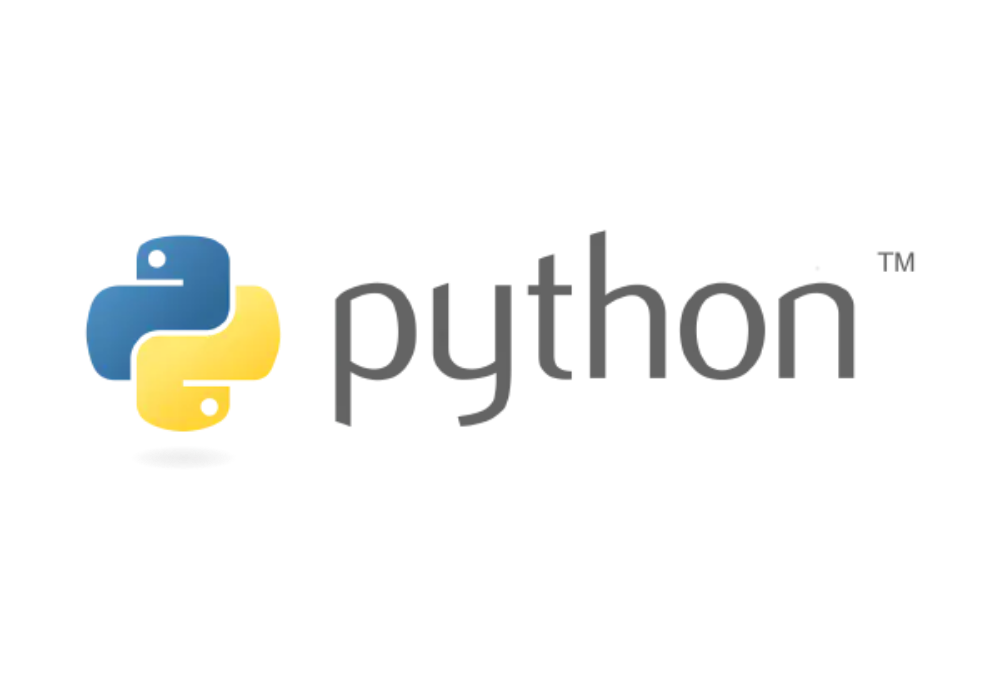Connect With Us:## Python101: 6. Modules and PackagesPython
by Admin/ on 04 Jul 2021

## Python101: 6. Modules and Packages

Modules and packages are the core of any large program, and even the Python installer itself is a package.

Focuses on common programming techniques related to modules and packages, such as how to organize packages, splitting large modules into multiple files, and creating namespace packages. Also gives tips for letting you customize your import statements.

Let’s start by explaining the concept between modules, packages and libraries.

• A module is a py file that defines functions, classes, variables, etc.
• package is a folder formed by aggregating multiple modules, which can be multiple py files, or nested folders
• Libraries are a reference to other programming languages, and are collections of code that perform certain functions, which in Python take the form of modules and packages

A module is actually a py file that encapsulates a set of functions; a package is a class of modules grouped together, and is a bit larger than the concept of a module; a library is a group of functions encapsulated by other programmers, and is generally a bit larger than the concept of a package.

Let’s separate the following.

## Module

From the above, we know that a module is a py file, a text file that stores a set of functions that we can use again to improve the reuse of code. We call this a py file a Python module. In other Python scripts, the defined Python module is loaded by import.

### Define and call Python modules

Let’s start by looking at how to define a Python module.

Define a hello.py module with the following content.

``````def sayhello( ):
print("Hello World!")
``````

Usually we use the import statement to introduce modules with the following syntax.

``````import module1[, module2[,... moduleN]]
``````

When the interpreter encounters an import statement, the module will be imported if it is in the current search path. The call is made using `module name. function name` to make the call

In the above example, we create a new `do.py` file to call the methods in the `hello.py` module.

The `do.py` file reads as follows.

``````# Import modules
import hello

# The functions included in the module can now be called
hello.sayhello()
``````

A module will only be imported once, no matter how many times you import it. this prevents the imported module from being executed over and over again.

Execute the shortcut ctrl+b on the do.py page and the console outputs: Hello World!

This is an example of a module definition and call, is not also very simple.

### from … import …

Modules provide namespace-like restrictions that allow Python to import specified symbols (variables, functions, classes, etc.) from a module into the current module. Once imported, these symbols can be used directly without prefixing the module name.

The syntax is as follows.

``````from modname import name1[, name2[, ... nameN]]
``````

For example, to import the sayhello function of the module hello, use the following statement.

``````## Direct import method
from hello import sayhello
sayhello()
``````

from … import * statement

It is also possible to import all the content of a module into the current namespace, simply by using the following declaration.

``````from modname import *
``````

This provides an easy way to import all items in a module.

We add another world method to hello.py.

``````def world():
print("Python World!")
``````

Introduce all the methods to be called in the do.py file.

``````## Import all methods
from hello import *
sayhello()
world()
``````

Output after execution.

``````Hello World!
Python World!
``````

This proves that both methods in the hello module can be called directly and are not recommended to be used too much in real projects.

## Package

A package is a higher-level abstraction of a module in Python. Simply put, Python allows users to treat directories as modules. In this way, the different module files in a directory become submodules within a “package”. In addition, package directories can have subdirectories under them, and those subdirectories can also be Python packages. This kind of hierarchy is very beneficial for module identification and management.

In particular, for some large Python toolkits, there can be hundreds or thousands of modules with different functions inside. In scientific computing, third-party tools such as SciPy, NumPy, Matplotlib, etc., are distributed in packages.

### Package Definition

Common package structures are as follows.

``````pakageName
-------__init__.py
-------moduleName1.py
-------moduleName2.py
------- ...
``````

The `__init__.py` file must exist under the package path.

Example.

We create a package of cal with a model of the calculator with the following structure.

``````cal
-------__init__.py
-------calculator.py
``````

The code for the calculator.py module is as follows.

``````def add(a,b) :
return a+b

def reduce(a,b) :
return a-b

def multiply(a,b) :
return a*b

def divide(a,b) :
return a/b
``````

### Using Python packages

The use of Python packages is similar to the use of modules, and the following is the syntax for importing them.

``````import package name. Package name. Module name
``````

For example, we import `calculator.py` in do.py

``````# Import package
import cal.calculator

# Methods for modules that use the package
``````

But the import call has a long registration, so you can use `from ... import ... ` statement to simplify things a bit.

``````# Import package
from cal import calculator

# Use the methods of the package's module
print(calculator.multiply(3,6))
``````

The effect will also be better when the package name is getting longer.

## Summary

This section introduces you to the use of Python modules and packages, which provide support for Python engineers. The proper use of modules and packages can constantly improve the efficiency of your code and allow for more standardized calls throughout your project.

Reference